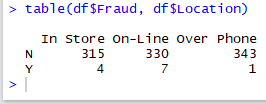# R: Table() Function in R

The table function in R is a great way to aggregate data for better evaluation and visualization. It is basically a pivot table like function for R. For those not familiar with pivot tables, I do have quick intro here: Excel: Intro to Pivot Tables

Building a table in R is easy. The syntax is simply table() with the name of variable you wish to aggregate inside the parenthesis.

In this example, I will be working with the imbalanced data set. You can download it here: imbalanced

Note: I’m importing data from an excel file in this example. You will need the readxl library installed to follow along. For instructions on installing libraries: R: Installing Packages with Dependencies

Let’s load the data and take a look at it:This data set has 1000 records, with 3 columns (or variables). The data represents a listing of possible fraudulent credit card transactions.

So what if you just want to know how many records are Fraud or Not-Fraud

Simply use the table() function with the variable df\$FraudNext we can try it with locationYou can even use more than one variable with the function: